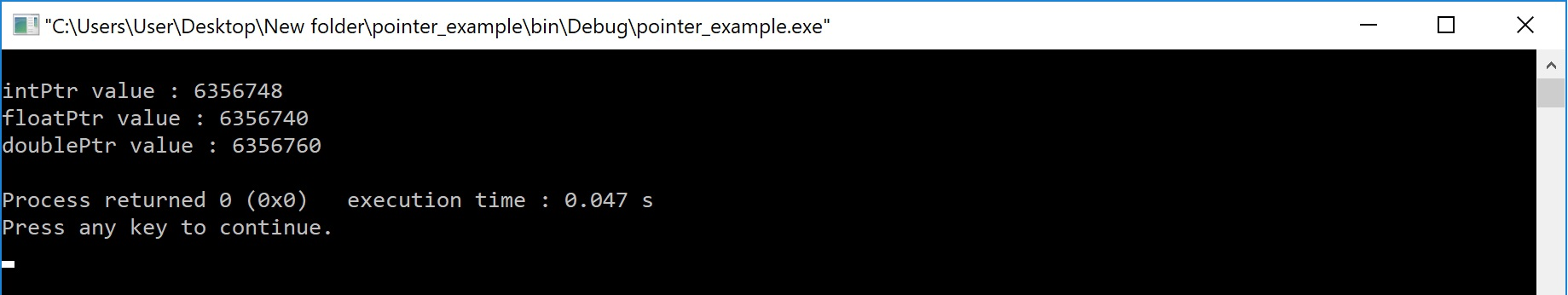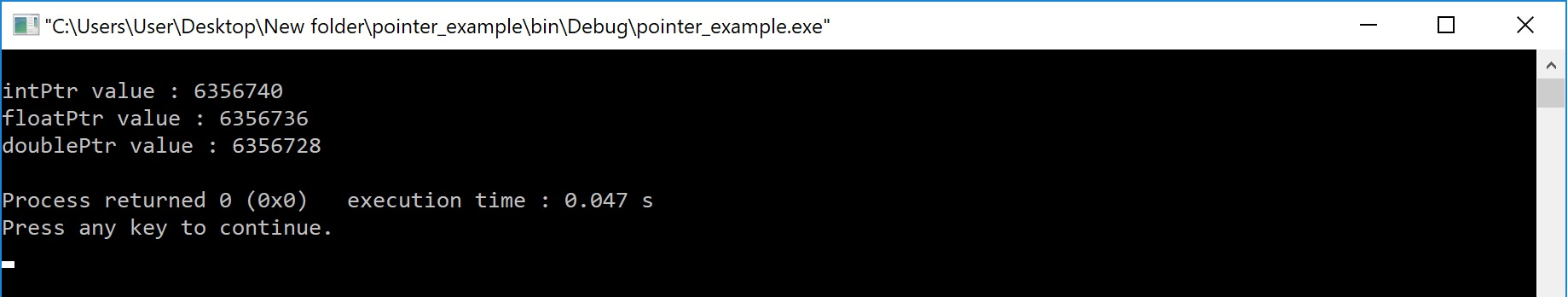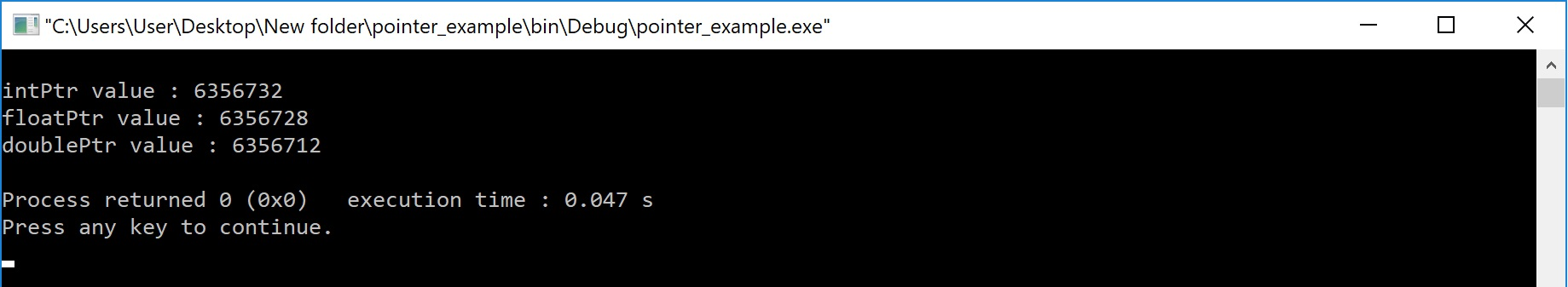The perfect place for easy learning...

×

# Pointers Arithmetic Operations in C

Pointer variables are used to store the address of variables. Address of any variable is an unsigned integer value i.e., it is a numerical value. So we can perform arithmetic operations on pointer values. But when we perform arithmetic operations on pointer variable, the result depends on the amount of memory required by the variable to which the pointer is pointing.

In the c programming language, we can perform the following arithmetic operations on pointers...

2. Subtraction
3. Increment
4. Decrement
5. Comparison

In the c programming language, the addition operation on pointer variables is calculated using the following formula...

# Example Program

``````void main()
{
int a, *intPtr ;
float b, *floatPtr ;
double c, *doublePtr ;
clrscr() ;
intPtr = &a ;  // Asume address of a is 1000
floatPtr = &b ;  // Asume address of b is 2000
doublePtr = &c ;  // Asume address of c is 3000

intPtr = intPtr + 3 ;  // intPtr = 1000 + ( 3 * 2 )
floatPtr = floatPtr + 2 ;  // floatPtr = 2000 + ( 2 * 4 )
doublePtr = doublePtr + 5 ;  // doublePtr = 3000 + ( 5 * 6 )

printf("intPtr value : %u\n", intPtr) ;
printf("floatPtr value : %u\n", floatPtr) ;
printf("doublePtr value : %u", doublePtr) ;

getch() ;
}```
```

Output:Subtraction Operation on Pointer

In the c programming language, the subtraction operation on pointer variables is calculated using the following formula...

# Example Program

``````void main()
{
int a, *intPtr ;
float b, *floatPtr ;
double c, *doublePtr ;
clrscr() ;
intPtr = &a ;  // Asume address of a is 1000
floatPtr = &b ;  // Asume address of b is 2000
doublePtr = &c ;  // Asume address of c is 3000

intPtr = intPtr - 3 ;  // intPtr = 1000 - ( 3 * 2 )
floatPtr = floatPtr - 2 ;  // floatPtr = 2000 - ( 2 * 4 )
doublePtr = doublePtr - 5 ;  // doublePtr = 3000 - ( 5 * 6 )

printf("intPtr value : %u\n", intPtr) ;
printf("floatPtr value : %u\n", floatPtr) ;
printf("doublePtr value : %u", doublePtr) ;

getch() ;
}```
```

Output:Increment & Decrement Operation on Pointer

The increment operation on pointer variable is calculated as follows...

# Example Program

``````void main()
{
int a, *intPtr ;
float b, *floatPtr ;
double c, *doublePtr ;
clrscr() ;
intPtr = &a ;  // Asume address of a is 1000
floatPtr = &b ;  // Asume address of b is 2000
doublePtr = &c ;  // Asume address of c is 3000

intPtr++ ;  // intPtr = 1000 + 2
floatPtr++ ;  // floatPtr = 2000 + 4
doublePtr++ ;  // doublePtr = 3000 + 6

printf("intPtr value : %u\n", intPtr) ;
printf("floatPtr value : %u\n", floatPtr) ;
printf("doublePtr value : %u", doublePtr) ;

getch() ;
}```
```

Output:The decrement operation on pointer variable is calculated as follows...

# Example Program

``````void main()
{
int a, *intPtr ;
float b, *floatPtr ;
double c, *doublePtr ;
clrscr() ;
intPtr = &a ;  // Asume address of a is 1000
floatPtr = &b ;  // Asume address of b is 2000
doublePtr = &c ;  // Asume address of c is 3000

intPtr-- ;  // intPtr = 1000 - 2
floatPtr-- ;  // floatPtr = 2000 - 4
doublePtr-- ;  // doublePtr = 3000 - 6

printf("intPtr value : %u\n", intPtr) ;
printf("floatPtr value : %u\n", floatPtr) ;
printf("doublePtr value : %u", doublePtr) ;

getch() ;
}```
```

Output:Comparison of Pointers

The comparison operation is perform between the pointers of same datatype only. In c programming language, we can use all comparison operators (relational operators) with pointers.

We can't perform multiplication and division operations on pointers.